• 作者：一流科技
• 发表时间：2021-02-25 14:15
• 来源：未知

Softmax操作是深度学习模型中最常用的操作之一。在深度学习的分类任务中，网络最后的分类器往往是Softmax + CrossEntropy的组合：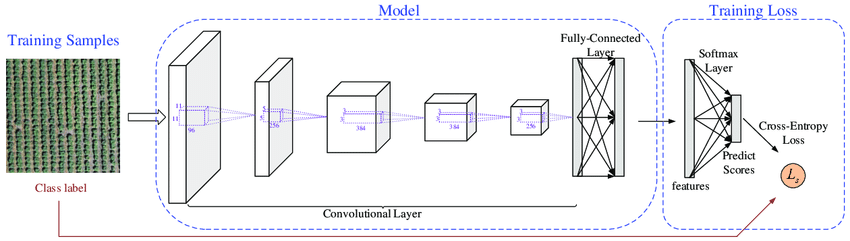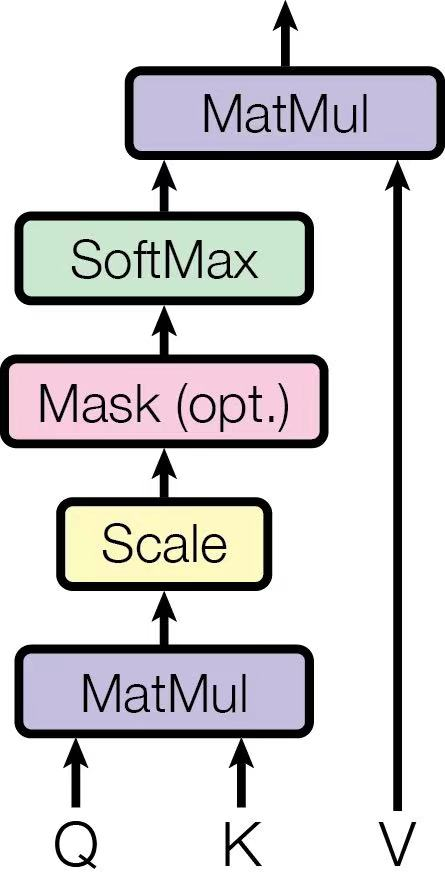### GPU基础知识与CUDA性能优化原则：

https://zhuanlan.zhihu.com/p/271740706

• GPU内存分为Global memory、Shared memory、Local memory三级。

• GPU最主要提供的是两种资源：计算资源显存带宽资源。如果我们能将这两种资源充分利用，且对资源的需求无法再降低，那么性能就优化到了极限，执行时间就会最短。在大多数情况下，深度学习训练中的GPU计算资源是被充分利用的，而一个GPU CUDA Kernel的优化目标就是尽可能充分利用显存带宽资源。

### Naive的Softmax实现：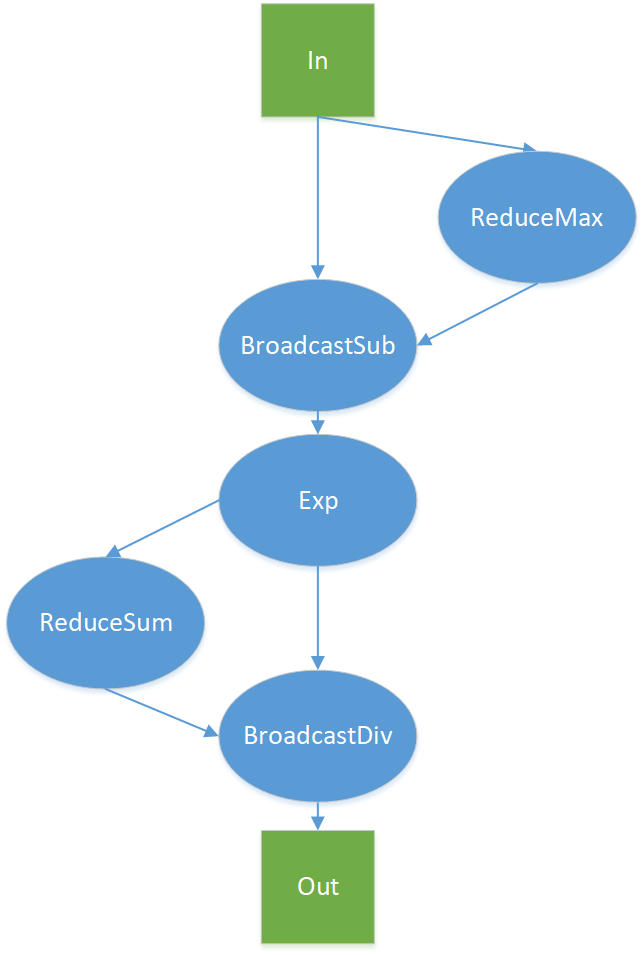• ReduceMax = `D + num_rows` （read 为 D， write 为 num_rows）
• BroadcaseSub = `2 * D + num_rows` （read 为 D + num_rows，write 为 D）
• Exp = `2 * D` （read 、write 均为D）
• ReduceSum = `D + num_rows`（read 为 D， write 为 num_rows）
• BroadcastDive = `2 * D + num_rows` （read 为 D + num_rows， write 为 D）

### OneFlow 与 cuDNN 的对比结果

Softmax Kernel Bandwidth：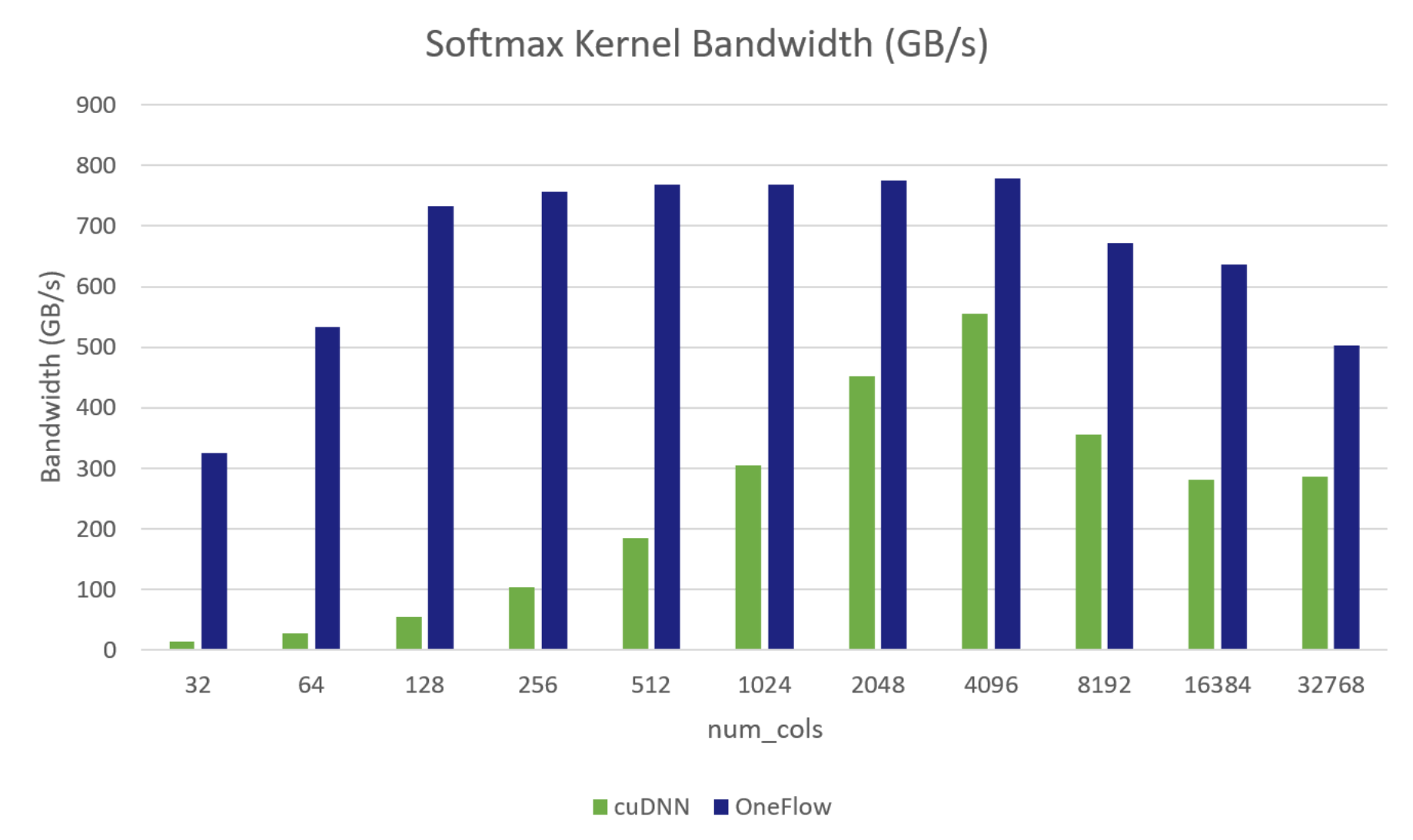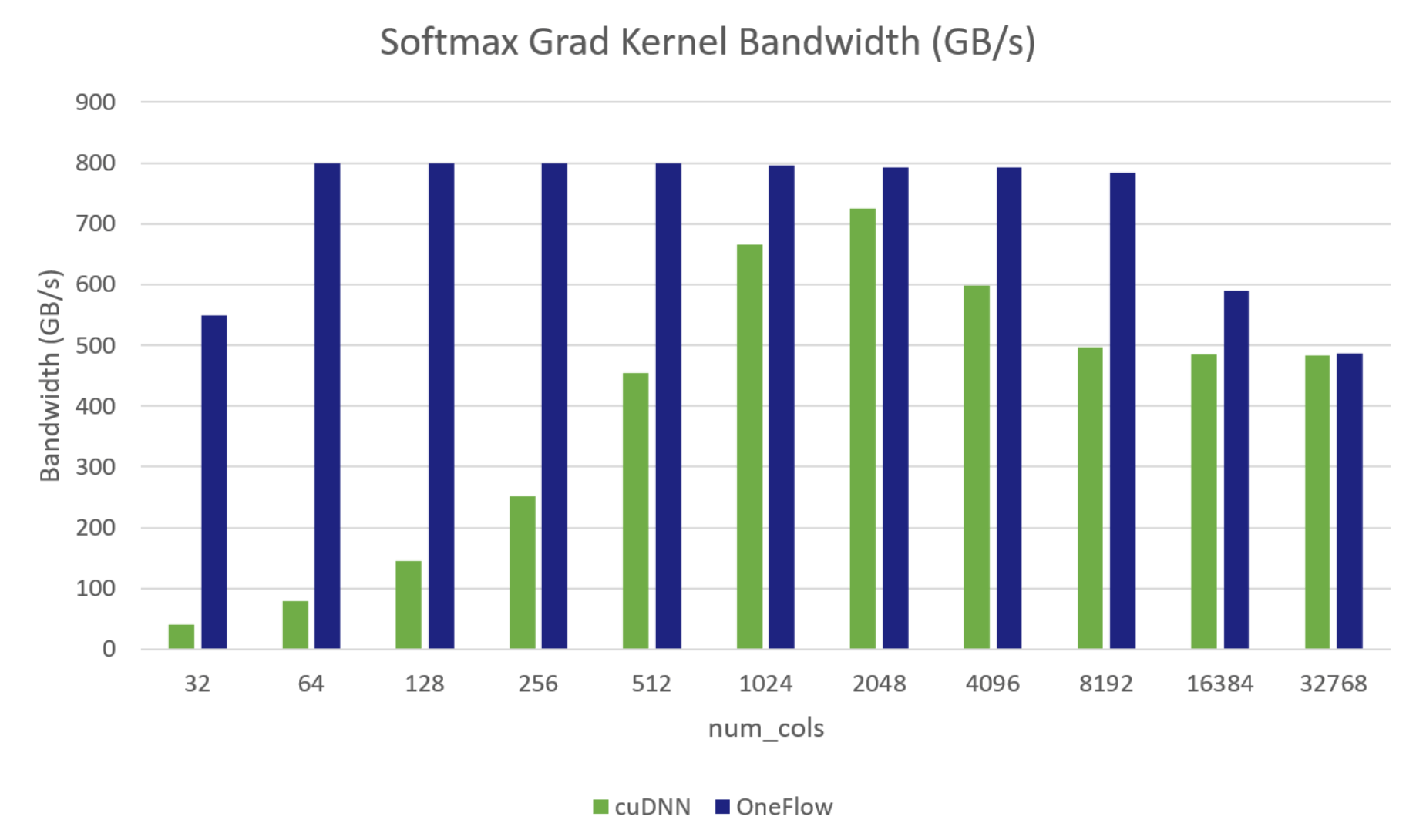### OneFlow深度优化Softmax CUDA Kernel的技巧

Softmax 函数的输入形状为:`(num_rows, num_cols)`，num_cols的变化，会对有效带宽产生影响；因为，没有一种 通用 的优化方法可以实现在所有 num_cols的情况下都是传输最优的。所以，在 OneFlow 中采用分段函数优化SoftmaxKernel：对于不同 num_cols范围，选择不同的实现，以在所有情况下都能达到较高的有效带宽。见 Optimize softmax cuda kernel

OneFlow 中分三种实现，分段对 softmax 进行优化：

(1) 一个 Warp 处理一行的计算，适用于 `num_cols <= 1024` 情况

(2) 一个 Block 处理一行的计算，借助 Shared Memory 保存中间结果数据，适用于需要的 Shared Memory 资源满足 Kernel Launch 的可启动条件的情况，在本测试环境中是 `1024 < num_cols <= 4096`

(3) 一个 Block 处理一行的计算，不使用 Shared Memory，重复读输入 x，适用于不支持(1)、（2)的情况

#### 实现1：每个Warp处理一行元素。

``````template<template<typename> typename ReductionOp, typename T>
__inline__ __device__ T WarpAllReduce(T val) {
val = ReductionOp<T>()(val, __shfl_xor_sync(0xffffffff, val, mask));
}
return val;
}
``````

``````template<typename T, int pack_size, int cols_per_thread, bool padding>
__global__ void SoftmaxWarpImpl(const int64_t rows, const int64_t cols, const T* x, T* y) {
static_assert(cols_per_thread % pack_size == 0, "");
constexpr int num_packs = cols_per_thread / pack_size;
using ComputeType = typename GetComputeType<T>::type;
const int global_warp_id = blockIdx.x * blockDim.y + threadIdx.y;
const int num_global_warp = gridDim.x * blockDim.y;
for (int64_t row = global_warp_id; row < rows; row += num_global_warp) {
const int64_t row_offset = row * cols;
const T* row_x = x + row_offset;
T* row_y = y + row_offset;
#pragma unroll
for (int pack_id = 0; pack_id < num_packs; ++pack_id) {
const int col = (pack_id * kWarpSize + lane_id) * pack_size;
if (!padding || col < cols) {
MultiFetch<T, ComputeType, pack_size>()(buf + pack_id * pack_size, row_x + col);
#pragma unroll
for (int i = 0; i < pack_size; ++i) {
}
} else {
#pragma unroll
for (int i = 0; i < pack_size; ++i) { buf[pack_id * pack_size + i] = -Inf<ComputeType>(); }
}
}
const ComputeType warp_max = WarpAllReduce<MaxOp, ComputeType>(thread_max);
#pragma unroll
for (int i = 0; i < cols_per_thread; ++i) {
buf[i] = exp(buf[i] - warp_max);
}
const ComputeType warp_sum = WarpAllReduce<SumOp, ComputeType>(thread_sum);
#pragma unroll
for (int i = 0; i < cols_per_thread; ++i) { buf[i] = buf[i] / warp_sum; }
#pragma unroll
for (int i = 0; i < num_packs; ++i) {
const int col = (i * kWarpSize + lane_id) * pack_size;
if (!padding || col < cols) {
MultiStore<ComputeType, T, pack_size>()(row_y + col, buf + i * pack_size);
}
}
}
}
``````

#### 实现2： 一个Block处理一行元素

``````template<template<typename> typename ReductionOp, typename T, int block_size>
__inline__ __device__ T BlockAllReduce(T val) {
typedef cub::BlockReduce<T, block_size> BlockReduce;
__shared__ typename BlockReduce::TempStorage temp_storage;
T result = BlockReduce(temp_storage).Reduce(val, ReductionOp<T>());
}
``````

``````template<typename T, int pack_size, int block_size>
__global__ void SoftmaxBlockSMemImpl(const int64_t rows, const int64_t cols, const T* x, T* y) {
using ComputeType = typename GetComputeType<T>::type;
extern __shared__ __align__(sizeof(ComputeType)) unsigned char shared_buf[];
auto* buf = reinterpret_cast<ComputeType*>(shared_buf);
assert(cols % pack_size == 0);
const int num_packs = cols / pack_size;
for (int64_t row = blockIdx.x; row < rows; row += gridDim.x) {
const int64_t row_offset = row * cols;
const T* row_x = x + row_offset;
T* row_y = y + row_offset;
for (int pack_id = tid; pack_id < num_packs; pack_id += block_size) {
ComputeType pack[pack_size];
MultiFetch<T, ComputeType, pack_size>()(pack, row_x + pack_id * pack_size);
#pragma unroll
for (int i = 0; i < pack_size; ++i) {
buf[i * num_packs + pack_id] = pack[i];
}
}
const ComputeType row_max = BlockAllReduce<MaxOp, ComputeType, block_size>(thread_max);
for (int col = tid; col < cols; col += block_size) {
const ComputeType exp_x = exp(buf[col] - row_max);
buf[col] = exp_x;
}
const ComputeType row_sum = BlockAllReduce<SumOp, ComputeType, block_size>(thread_sum);
for (int pack_id = tid; pack_id < num_packs; pack_id += block_size) {
ComputeType pack[pack_size];
#pragma unroll
for (int i = 0; i < pack_size; ++i) {
pack[i] = buf[i * num_packs + pack_id] / row_sum;
}
MultiStore<ComputeType, T, pack_size>()(row_y + pack_id * pack_size, pack);
}
}
}

``````

#### 实现3：一个Block处理一行的元素，不使用Shared Memory，重复读输入x

``````template<typename T, int pack_size, int block_size>
__global__ void SoftmaxBlockUncachedImpl(const int64_t rows, const int64_t cols, const T* x, T* y) {
using ComputeType = typename GetComputeType<T>::type;
assert(cols % pack_size == 0);
const int num_packs = cols / pack_size;
for (int64_t row = blockIdx.x; row < rows; row += gridDim.x) {
const int64_t row_offset = row * cols;
const T* row_x = x + row_offset;
T* row_y = y + row_offset;
for (int pack_id = tid; pack_id < num_packs; pack_id += block_size) {
ComputeType pack[pack_size];
MultiFetch<T, ComputeType, pack_size>()(pack, row_x + pack_id * pack_size);
#pragma unroll
for (int i = 0; i < pack_size; ++i) { thread_max = max(thread_max, pack[i]); }
}
const ComputeType row_max = BlockAllReduce<MaxOp, ComputeType, block_size>(thread_max);
for (int pack_id = tid; pack_id < num_packs; pack_id += block_size) {
ComputeType pack[pack_size];
MultiFetch<T, ComputeType, pack_size>()(pack, row_x + pack_id * pack_size);
#pragma unroll
for (int i = 0; i < pack_size; ++i) { thread_sum += exp(pack[i] - row_max); }
}
const ComputeType row_sum = BlockAllReduce<SumOp, ComputeType, block_size>(thread_sum);
for (int pack_id = tid; pack_id < num_packs; pack_id += block_size) {
ComputeType pack[pack_size];
MultiFetch<T, ComputeType, pack_size>()(pack, row_x + pack_id * pack_size);
#pragma unroll
for (int i = 0; i < pack_size; ++i) { pack[i] = exp(pack[i] - row_max) / row_sum; }
MultiStore<ComputeType, T, pack_size>()(row_y + pack_id * pack_size, pack);
}
}
}
``````

### 公共优化技巧：

1、将 Half 类型 pack 成 Half2 进行存取，在不改变延迟情况下提高指令吞吐，类似 CUDA template for element-wise kernels 的优化

2、Shared Memory 中的 Bank Conflicts

CUDA 将 Shared Memory 按照4字节或8字节大小划分到32个 bank 中，对于 Volta 架构是4字节，这里以4字节为例，如下图所示，0-128 bytes地址分别在bank 0-31中，128-256也分别在 bank0-31中。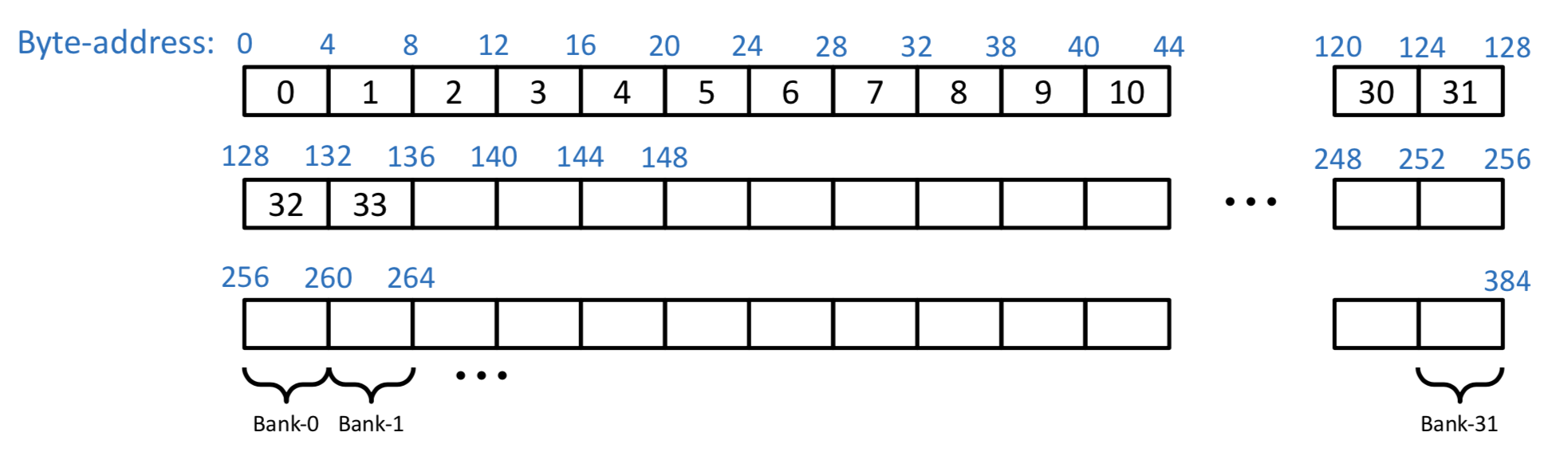：此图及以下 `Bank Conflicts` 图片来自VOLTA Architecture and performance optimization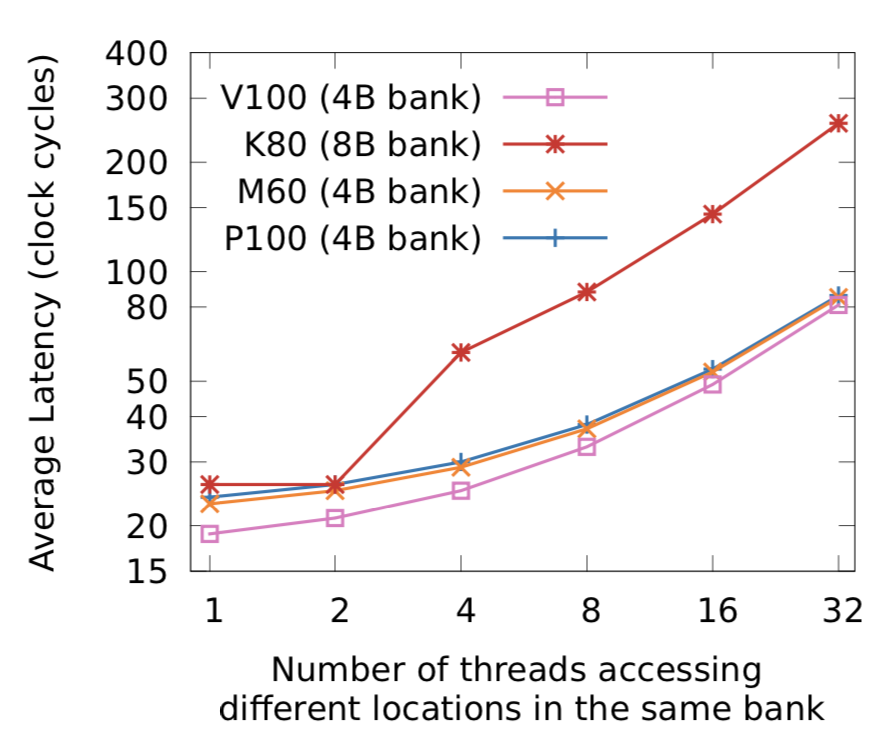Bank Conflicts 的几种情况：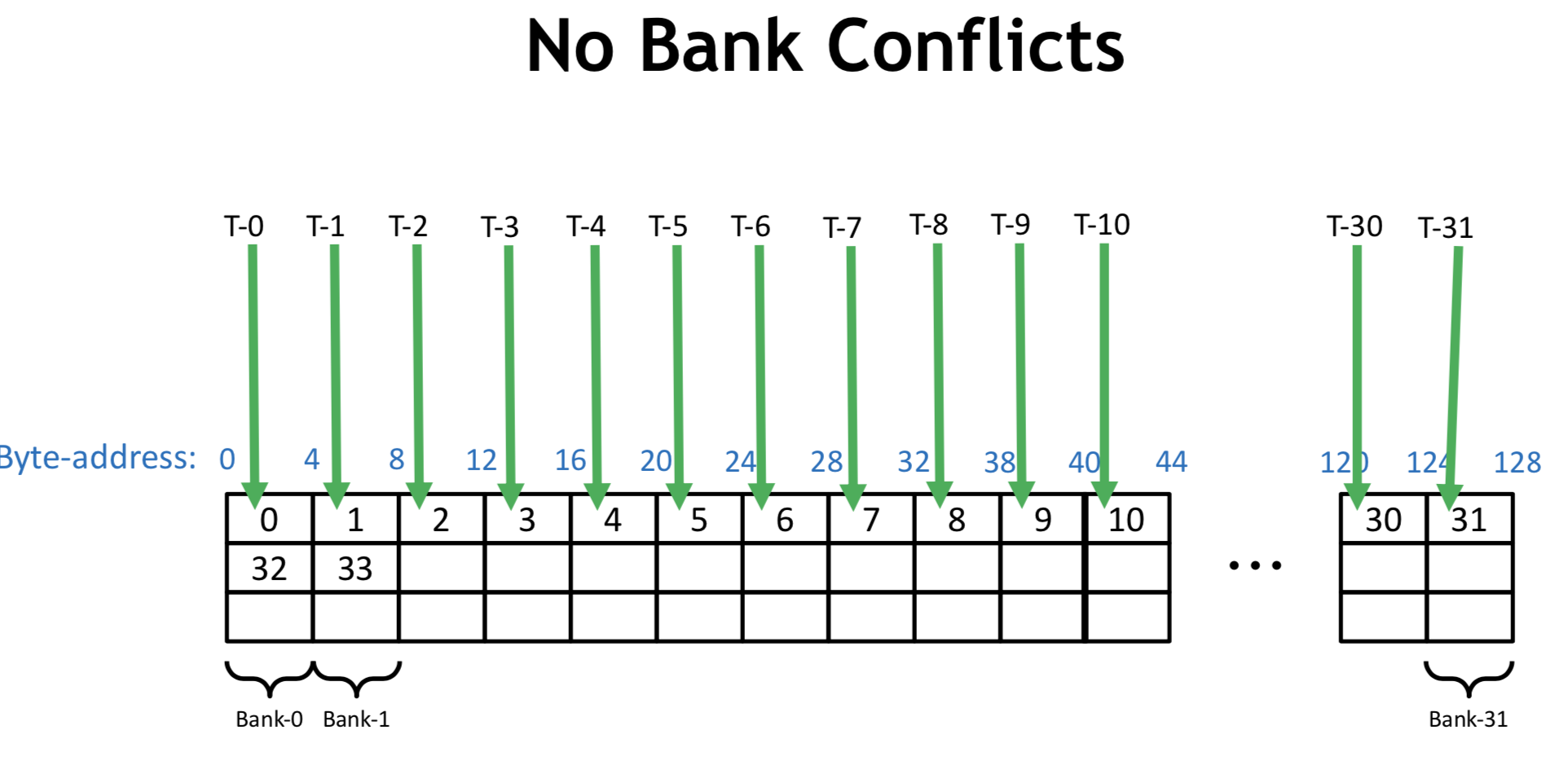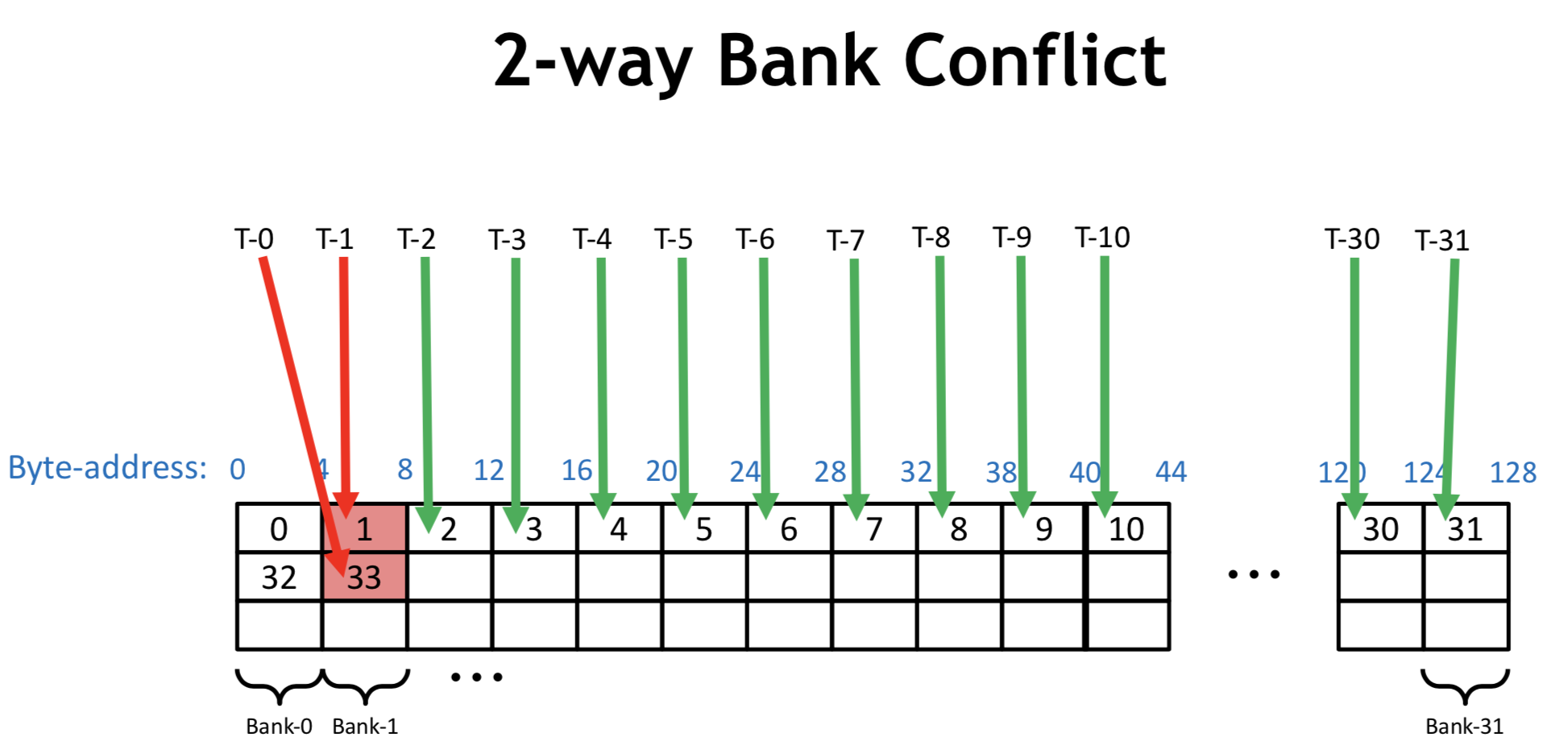``````#pragma unroll
for (int j = 0; j < pack_size; ++j) {
buf[pack_id * pack_size * j] = pack[j];
``````#pragma unroll